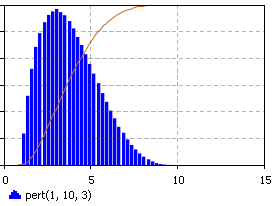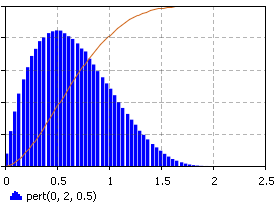AnyLogic

# pert

The PERT distribution is a continuous distribution bounded on both sides. Being an alternative distribution to the Triangular, it has the same three inputs, Minimum, Most Likely, and Maximum, but is a smooth curve that puts less emphasis on extreme values. The PERT distribution is often used in risk analysis applications, e.g. in Monte Carlo simulations to assess cost and project duration risks.

### Samples (PDF, CDF)### pert(double min, double max, double mode)

Description
Generates a sample of the PERT distribution.
Parameters
Name Type Description
min double the minimum x value
max double the maximum x value
mode double the most likely x value
Result
Type Description
double the generated sample

### pert(double min, double max, double mode, java.util.Random r)

Description
Generates a sample of the PERT distribution using the specified random number generator.
Parameters
Name Type Description
min double the minimum x value
max double the maximum x value
mode double the most likely x value
r java.util.Random the random number generator
Result
Type Description
double the generated sample